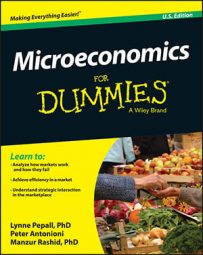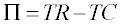##### Microeconomics For DummiesThe most global view of a company is in terms of its total costs (TC), which economists use quite simply to arrive at a number for total profits (you don't need to worry about gross or net terms here). Total costs are simply the overall cost of making a product and serving it to a market after all those relevant cost elements are accounted for.

When talking about the types of cost a firm faces, economists start with the biggest and least detailed view of costs and then break them down in ways that make sense for economic decision-making.

If you start with a number for total revenue received (TR) and then subtract all those costs, you arrive at a figure for profit. We write that as an equation here:The Greek symbol pi in the equation stands for profit, so that economists don't get confused with price (generally denoted with a P).

When you take the view of firm owner, you may want to start looking at aspects of the total cost a little more closely. The simplest way to break down total costs is into two categories:

• Costs that depend on the amount you've produced: These include all things that are ongoing in the sense that the more you produce — or for a service, the more activity you undergo — the more you pay, which means things such as costs of each input used or the cost of utilities. These are called variable costs, and economists usually denote them VC when talking about variable costs as a component of total costs.

• Costs that don't depend on the amount you've produced: These include all the elements that your firm would have to pay regardless of how many items it produces. These may be the cost of premises, such as rents, or licenses if needed, or even the cost of advertising that the firm has to buy to let people know about its business. Economists call these fixed costs and usually abbreviate them FC.

Together, the two types of cost must sum to the total cost at which the firm operates, so you can say:

TC = FC + VC

Here's an example: A bicycle manufacturer makes bikes using labor, materials, and utilities costing \$10 a bike. Assuming that she makes 100 bikes in a production period, the variable costs (VC) she faces will be 100 bikes times \$10 a bike, which equals \$1,000. However, in order to do this she needs a factory with the capacity to make the bikes: That factory costs \$10,000 to rent per period. So her total costs are fixed costs (FC) of \$10,000 plus the VC of \$1,000, which equals \$11,000 total costs.

Here are two important points to bear in mind:

• You have to pay fixed costs even if you produce absolutely no output whatsoever. If, for instance, you take out a rental contract for an office, you have to pay the rent irrespective of whether you produce anything at all.

• Variable costs depend on the amount of output you produce. If you have a pizza restaurant and you make no pizzas, you don't need any flour, tomatoes, or even power for a pizza oven. But the more pizzas you make, the more you spend on these inputs.